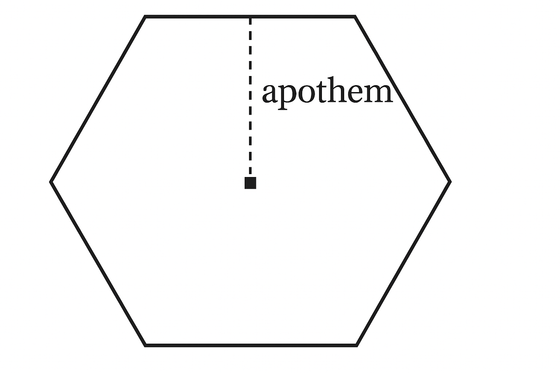index: click on a letter A B C D E F G H I J K L M N O P Q R S T U V W X Y Z A to Z index index: subject areas numbers & symbols sets, logic, proofs geometry algebra trigonometry advanced algebra & pre-calculus calculus advanced topics probability & statistics real world applications multimedia entrieswww.mathwords.com about mathwords website feedback

 Apothem The line segment from the center of a regular polygon to the midpoint of a side, or the length of this segment. Same as the inradius; that is, the radius of a regular polygon's inscribed circle. Note: Apothem is pronounced with the emphasis on the first syllable with the a pronounced as in apple (A-puh-thum).